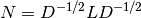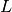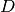Warning

This documents an unmaintained version of NetworkX. Please upgrade to a maintained version and see the current NetworkX documentation.

# normalized_laplacian_matrix¶

normalized_laplacian_matrix(G, nodelist=None, weight='weight')[source]

Return the normalized Laplacian matrix of G.

The normalized graph Laplacian is the matrixwhereis the graph Laplacian andis the diagonal matrix of node degrees.

Parameters: G (graph) – A NetworkX graph nodelist (list, optional) – The rows and columns are ordered according to the nodes in nodelist. If nodelist is None, then the ordering is produced by G.nodes(). weight (string or None, optional (default=’weight’)) – The edge data key used to compute each value in the matrix. If None, then each edge has weight 1. N – The normalized Laplacian matrix of G. NumPy matrix

Notes

For MultiGraph/MultiDiGraph, the edges weights are summed. See to_numpy_matrix for other options.

If the Graph contains selfloops, D is defined as diag(sum(A,1)), where A is the adjacency matrix .

References

  Fan Chung-Graham, Spectral Graph Theory, CBMS Regional Conference Series in Mathematics, Number 92, 1997.
  Steve Butler, Interlacing For Weighted Graphs Using The Normalized Laplacian, Electronic Journal of Linear Algebra, Volume 16, pp. 90-98, March 2007.